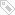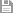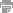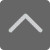# 高中数学知识点：数列

2018-11-14 08:02:39 　来源：网络整理

高中数学知识点：数列！我们在学习高中数学的时候，除了上课认真听老师讲解外，学习方法，学习习惯也很重要，只要认真努力，数学成绩提高是很容易的。下面就是小编特意为同学们整理的高中数学知识点：数列，希望对大家有所帮助。

数列的相关概念

1.数列概念

①数列是一种特殊的函数。其特殊性主要表现在其定义域和值域上。数列可以看作一个定义域为正整数集N*或其有限子集{1，2，3，…，n}的函数，其中的{1，2，3，…，n}不能省略。

②用函数的观点认识数列是重要的思想方法，一般情况下函数有三种表示方法，数列也不例外，通常也有三种表示方法：a.列表法;b。图像法;c.解析法。其中解析法包括以通项公式给出数列和以递推公式给出数列。

③函数不一定有解析式，同样数列也并非都有通项公式。

等差数列

1.等差数列通项公式

an=a1+(n-1)d

n=1时a1=S1

n≥2时an=Sn-Sn-1

an=kn+b(k,b为常数)推导过程：an=dn+a1-d令d=k，a1-d=b则得到an=kn+b

2.等差中项

由三个数a，A，b组成的等差数列可以堪称较简单的等差数列。这时，A叫做a与b的等差中项(arithmeticmean)。

有关系：A=(a+b)÷2

3.前n项和

倒序相加法推导前n项和公式：

Sn=a1+a2+a3+·····+an

=a1+(a1+d)+(a1+2d)+······+[a1+(n-1)d]①

Sn=an+an-1+an-2+······+a1

=an+(an-d)+(an-2d)+······+[an-(n-1)d]②

由①+②得2Sn=(a1+an)+(a1+an)+······+(a1+an)(n个)=n(a1+an)

∴Sn=n(a1+an)÷2

等差数列的前n项和等于首末两项的和与项数乘积的一半：

Sn=n(a1+an)÷2=na1+n(n-1)d÷2

Sn=dn2÷2+n(a1-d÷2)

亦可得

a1=2sn÷n-an=[sn-n(n-1)d÷2]÷n

an=2sn÷n-a1

有趣的是S2n-1=(2n-1)an，S2n+1=(2n+1)an+1

4.等差数列性质

一、任意两项am，an的关系为：

an=am+(n-m)d

它可以看作等差数列广义的通项公式。

二、从等差数列的定义、通项公式，前n项和公式还可推出：

a1+an=a2+an-1=a3+an-2=…=ak+an-k+1，k∈N*

三、若m，n，p，q∈N*，且m+n=p+q，则有am+an=ap+aq

四、对任意的k∈N*，有

Sk，S2k-Sk，S3k-S2k，…，Snk-S(n-1)k…成等差数列。

等比数列

1.等比中项

如果在a与b中间插入一个数G，使a，G，b成等比数列，那么G叫做a与b的等比中项。

有关系：

注：两个非零同号的实数的等比中项有两个，它们互为相反数，所以G²=ab是a,G,b三数成等比数列的必要不充分条件。

2.等比数列通项公式

an=a1*q’(n-1)(其中首项是a1，公比是q)

an=Sn-S(n-1)(n≥2)

前n项和

当q≠1时，等比数列的前n项和的公式为

Sn=a1(1-q’n)/(1-q)=(a1-a1*q’n)/(1-q)(q≠1)

当q=1时，等比数列的前n项和的公式为

Sn=na1

3.等比数列前n项和与通项的关系

an=a1=s1(n=1)

an=sn-s(n-1)(n≥2)

4.等比数列性质

(1)若m、n、p、q∈N*，且m+n=p+q，则am·an=ap·aq;

(2)在等比数列中，依次每k项之和仍成等比数列。

(3)从等比数列的定义、通项公式、前n项和公式可以推出：a1·an=a2·an-1=a3·an-2=…=ak·an-k+1，k∈{1,2,…，n}

(4)等比中项：q、r、p成等比数列，则aq·ap=ar²，ar则为ap，aq等比中项。

记πn=a1·a2…an，则有π2n-1=(an)2n-1，π2n+1=(an+1)2n+1

另外，一个各项均为正数的等比数列各项取同底指数幂后构成一个等差数列;反之，以任一个正数C为底，用一个等差数列的各项做指数构造幂Can，则是等比数列。在这个意义下，我们说：一个正项等比数列与等差数列是“同构”的。

(5)等比数列前n项之和Sn=a1(1-q’n)/(1-q)

(6)任意两项am，an的关系为an=am·q’(n-m)

(7)在等比数列中，首项a1与公比q都不为零。

注意：上述公式中a’n表示a的n次方。

以上就是小编特意为大家整理的高中数学知识点：数列，同学们学习上如果有任何疑问，可以拨打免费咨询热线：4000—121—121，那里有专业的教育规划师会为您解答。标签：保存　｜打印　｜关闭